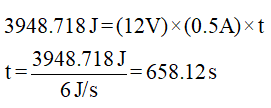# A sample of 10 g of liquid benzene at its boiling point is vaporised using a 12 V, 0.5 A electric supply. The normal boiling point and latent heat of vaporisation of benzene are 353.2 K and 30.8 × 10³ kJ/kmol respectively. Determine: (a) the change in internal energy, (b) the time required for complete vaporisation.

Question-AnswerCategory: Fluid MechanicsA sample of 10 g of liquid benzene at its boiling point is vaporised using a 12 V, 0.5 A electric supply. The normal boiling point and latent heat of vaporisation of benzene are 353.2 K and 30.8 × 10³ kJ/kmol respectively. Determine: (a) the change in internal energy, (b) the time required for complete vaporisation.

A sample of 10 g of liquid benzene at its boiling point is vaporised using a 12 V, 0.5 A electric supply. The normal boiling point and latent heat of vaporisation of benzene are 353.2 K and 30.8 × 10³ kJ/kmol respectively. Determine: (a) the change in internal energy, (b) the time required for complete vaporisation.

(a) The change in internal energy for the benzene is to be determined.

The equation for enthalpy and internal energy change is given as,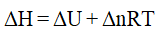……. (1)
H = Enthalpy of the reaction
U = Internal energy of the reaction
R = Universal gas constant
T = Temperature
n = Number of moles
The vaporization of the benzene is given as,The data available is,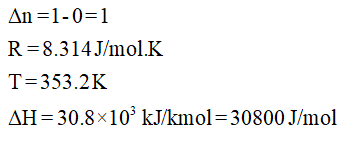Substitute the above data in equation (1),(b) The time required for the complete vaporization of the benzene is to be determined.

The energy required for complete vaporization of the benzene is,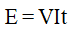……. (2)
E = Energy required
V = Voltage
I = Current
t = Time required
Let number of moles of benzene for complete vaporization = n1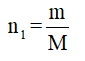……. (3)
m = Mass of the benzene given
M = Molar mass of the benzene

m = 10 g
M = 78 g/mol

Substitute the above data in equation (3),Now, the energy required is given as,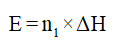……. (4)
Substitute change in enthalpy from part (a) and n1 in equation (4),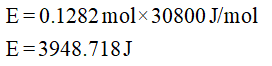Thus, the data now is,
E = 3948.718 J
V = 12 V
I = 0.5 A
Substitute the above data in equation (2),